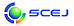## \$B9V1i%W%m%0%i%`!J2q>l!&F|DxJL!K(B

English page

### B \$B2q>l!&Bh(B 2 \$BF|(B

\$B:G=*99?7F|;~!'(B2014-11-19 12:59:10
\$B9V1i(B
\$B;~9o(B
\$B9V1i(B
\$BHV9f(B
\$B9V1iBjL\!?H/I=\$B%-!<%o!<%I(B\$BJ,N`(B
\$BHV9f(B
\$B\$BHV9f(B
\$B%7%s%]%8%&%`(B <\$B4D6-%(%M%k%.!<%7%s%]%8%&%`!c>7BT!&0MMj9V1i!"0lHL8xJg!d(B>
(9:20\$B!A(B12:00) (\$B:BD9(B \$B4_(B \$BH%!&D+7'(B \$BM52p(B)
9:20\$B!A(B 9:40B202\$BC4;}(BNi\$B?(G^>e\$G\$N%a%?%s\$NFs;@2=C:AG2~
(\$B?@8MBg1!9)(B) \$B!{(B(\$B3X(B)\$B;086(B \$BD>Li(B\$B!&(B \$BKLLn(B \$BM*4p(B\$B!&(B (\$B3X(B)\$B23K\(B \$BFF;K(B\$B!&(B (\$B@5(B)\$BC+20(B \$B7\$B!&(B (\$B@5(B)\$B;T66(B \$BM40l(B\$B!&(B (\$B@5(B)\$B@>;3(B \$B3P(B
Reforming
Support Basicity
Carbon deposition
S-173
9:40\$B!A(B 10:00B203\$B2D;k8w(BLED\$B>H
(\$B5~Bg4D2J%;(B) \$B!{(B(\$B@5(B)\$BCf@n(B \$B9@9T(B\$B!&(B (\$B5~Bg9)(B) (\$B3X(B)\$BA0@n(B \$B=_(B\$B!&(B (\$B@5(B)\$BA0(B \$B0lW"(B
1,4-dioxane
Fenton oxidation
visible LED
S-1108
10:00\$B!A(B 10:20B204\$B1v2=%+%k%7%&%`(B/\$BCf6u%7%j%+%3%s%]%8%C%HFb\$NG.J*
(\$B?@8MBg1!(B) \$B!{(B(\$B3X(B)\$B_7(B \$B>04u(B\$B!&(B (\$B%U%!%s%/%7%g%J%k%U%k%\$%C%I(B) (\$B@5(B)\$BF#2,(B \$B7C;R(B\$B!&(B (\$B?@8MBg<+(B) (\$B@5(B)\$BF|=P4V(B \$B\$k\$j(B\$B!&(B (\$B?@8MBg1!9)(B) (\$B@5(B)\$BNkLZ(B \$BMN(B\$B!&(B (\$B@5(B)\$B8VED(B \$B1YG7(B
heat and mass transfer
hollow silica particles
heat pump
S-1110
10:20\$B!A(B 10:40B205\$B>x5\$%(%8%'%/%?\$rMQ\$\$\$?2
(\$BJ<8K8)Bg(B) \$B!{(B(\$B@5(B)\$B0KF#(B \$BOB9((B\$B!&(B (\$B?@8MBg(B) \$B0f86(B \$B0l9b(B\$B!&(B (\$B%F%/%N%W%i%s(B) \$B_70f(B \$B@5OB(B\$B!&(B (\$B?@8M9b@l(B) \$B@VBP(B \$B=(L@(B\$B!&(B \$BNkLZ(B \$BN45/(B\$B!&(B (\$B4D8&(B) \$B:49B(B \$B?.0l(B\$B!&(B (\$BJ<8K8)Bg(B) \$B7'4](B \$BGn<"(B
Anaerobic digestion
Steam ejector
Decompressing hydrothermal treatment
S-113
10:40\$B!A(B 11:00B206\$B=E
(\$B5~Bg9)(B) \$B!{(B(\$B@5(B)\$BiCED(B \$BN40l(B\$B!&(B (\$B3X(B)\$B>.;^(B \$BM44x(B\$B!&(B (\$B3X(B)\$BB<>e(B \$B>08c(B\$B!&(B (\$B@5(B)\$B2O@%(B \$B85L@(B\$B!&(B (\$B5~Bg%(%MM}(B) (\$B@5(B)\$B;01:(B \$B9'0l(B
co-processing
heavy oils
S-146
11:00\$B!A(B 11:20B207\$B%F%l%U%?%k;@N3;R\$N>WFMGK2u@-\$K5Z\$\\$97k>=;R%5%\$%:\$N1F6A(B
(\$BJ<8K8)Bg1!9)(B) \$B!{(B(\$B@5(B)\$BNkLZ(B \$BF;N4(B\$B!&(B \$B5H0f(B \$B6598(B\$B!&(B (\$B@5(B)\$BHSB<(B \$B7r\$B!&(B (\$B@5(B)\$B:4F#:,(B \$BBg;N(B
Collision Destructivity
Terephthalic Acid Particle
Crystallite Size
S-164
11:20\$B!A(B 12:00B208[\$B0MMj9V1i(B] \$B6/M6EEBN%J%N%W%l!<%H\$NAO@=\$H\$=\$N%^%k%A%U%'%m%\$%C%/1~MQ(B
(\$BJ<8K8)Bg(B) \$B!{(B(\$B@5(B)\$B>.=.(B \$B@5J8(B
_
S-1120
12:00\$B!A(B 13:00\$BCk5Y\$_(B

\$B9V1iH/I=%W%m%0%i%`(B
\$B2=3X9)3X2q(B \$BI1O)Bg2q(B 2014(C) 2014 \$B8x1W
Most recent update: 2014-11-19 12:59:10
E-mail: inquiry-ks2014www3.scej.org
This page was generated byeasp 2.35; proghtml 2.35 (C)1999-2014 kawase